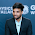Structural Analysis MCQ Test - Set 02 - ObjectiveBooks

# Practice Test: Question Set - 02

1. For a symmetrical two hinged parabolic arch, if one of the supports settles horizontally, then the horizontal thrust
(A) Is increased
(B) Is decreased
(C) Remains unchanged
(D) Becomes zero

2. To generate the jth column of the flexibility matrix
(A) A unit force is applied at coordinate j and the displacements are calculated at all coordinates
(B) A unit displacement is applied at coordinate j and the forces are calculated at all coordinates
(C) A unit force is applied at coordinate j and the forces are calculated at all coordinates
(D) A unit displacement is applied at coordinate ‘j’ and the displacements are calculated at all coordinates

3. A load 'W is moving from left to right support on a simply supported beam of span ‘T’. The maximum bending moment at 0.41 from the left support is
(A) 0.16 WL
(B) 0.20 WL
(C) 0.24 WL
(D) 0.25 WL

4. In the displacement method of structural analysis, the basic unknowns are
(A) Displacements
(B) Force
(C) Displacements and forces
(D) None of the above

5. A rigid-jointed plane frame is stable and statically determinate if
(A) (m + r) = 2j
(B) (m + r) = 3j
(C) (3m + r) = 3j
(D) (m + 3r) = 3j
Where m is number of members, r is reaction components and j is number of joints

6. In moment distribution method, the sum of distribution factors of all the members meeting at any joint is always
(A) Zero
(B) Less than 1
(C) 1
(D) Greater than 1

7. When a uniformly distributed load, shorter than the span of the girder, moves from left to right, then the conditions for maximum bending moment at a section is that
(A) The head of the load reaches the section
(B) The tail of the load reaches the section
(C) The load position should be such that the section divides it equally on both sides
(D) The load position should be such that the section divides the load in the same ratio as it divides the span

8. The width of the analogous column in the method of column analogy is
(A) 2/EI
(B) 1/EI
(C) 1/2 EI
(D) 1/4 EI

9. Muller Breslau's principle for obtaining influence lines is applicable to
(i) Trusses
(ii) Statically determinate beams and frames
(iii) Statically indeterminate structures, the material of which is elastic and follows Hooke's law
(iv) Any statically indeterminate structure
The correct answer is
(A) (i), (ii) and (iii)
(B) (i), (ii) and (iv)
(C) (i) and (ii)
(D) Only (i)

10. In column analogy method, the area of an analogous column for a fixed beam of span ‘L’ and flexural rigidity ‘EI’ is taken as
(A) L/EI
(B) L/2EI
(C) L/3EI
(D) L/4EI

Show and hide multiple DIV using JavaScript View All Answers

Blogger Comment
Facebook Comment

#### 1 blogger:

1.